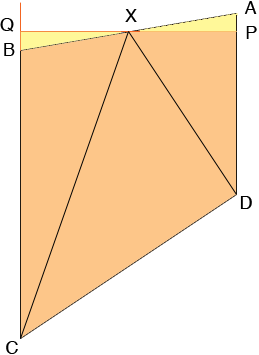SEARCH HOMEMath Central Quandaries & QueriesQuestion from James, a student: Hi, The sides BC and AD of a quadrilateral ABCD are parallel. X is the midpoint of AB. The area of ABCD is Y. Find the area of the triangle CXD in the terms of Y. Thank you so much for your help JamesHi James,

I drew a diagram and then drew a line through X, perpendicular to AD and meeting AD at P and meeting CB at Q.Since AD and BC are parallel angle XPA and angle XQB are congruent and therefore both are right angles. It follows that triangles AXP and XQB are similar and since |BX| = |XA| these two triangles are congruent and therefore have the same area. Thus the area of the quadrilateral PQCD is Y. Thus you can ask your original question of the quadrilateral PQCD where angles DPQ and PQC are right angles.

Does this help?
HarleyMath Central is supported by the University of Regina and The Pacific Institute for the Mathematical Sciences.